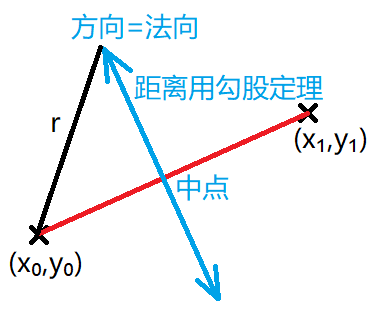🏆 第 189 场力扣周赛

16

#### C:收藏清单

1. 先对每个人的心仪公司排序：favoriteCompanies 的 size 小的排在前面，size 相同则按里面的每个 company 字典序排序
2. 进过排序，后者的 favoriteCompanies list 不可能是前者的子集，因此只需要判断前者是否为后者的子集，即对于第 i 人，判断是否为 [i+1, n-1] 人中任意一人的子集
3. 现在问题转移为，判断 favoriteCompanies[i] 是否为 favoriteCompanies[j] 的子集：由于每个人的 favoriteCompanies 都经过排序，用 two pointer 在两个序列中扫一遍即可
4. 时间复杂度为 O(n * n * m * len)，n 为人数，m 为一个人的 favoriteCompanies 个数，len 为一个公司名的字符串长度

## D:圆形靶内的最大飞镖数量

1. 首先枚举一个点作为圆心 A，然后再循环其它 N-1 个点，设当前循环到的点为 B

2. 用 atan2 函数求直线 BA 的极角 th，为方便极角排序，对于负角要 + 2pi

3. 求圆 A, B 的交点与 AB 的夹角 ph = acos(D / 2)，D = dist(A, B)

4. 计算圆 B 覆盖圆 A 的弧的始末极角 th - ph + 2pi 和 th + ph + 2pi，为了避免负角，每个极角均加2pi。同时加上标记区分始末点

5. 对这 2 * n 个始末点排序，扫描一遍，得到覆盖次数最多的弧的被覆盖次数，更新答案

7

6

#### 圆形靶内的最大飞镖数量class Solution {
//获得给定两点坐标和半径，获得圆心。
public double[] getCenter(int x0,int y0,int x1,int y1,int r){
int dx=x1-x0,dy=y1-y0;
double dist=Math.sqrt(dx*dx+dy*dy);
if(dist>2*r)
return new double; //不可能有圆心
double midx=(x0+x1)/2.0,midy=(y0+y1)/2.0;
if(dist==2*r)
return new double[]{midx,midy}; //圆心只有1种可能
double offset=Math.sqrt(r*r-dist*dist/4);
double offx=-dy/dist,offy=dx/dist;
return new double[]{midx+offx*offset,midy+offy*offset,midx-offx*offset,midy-offy*offset}; //圆心有2种可能
}

public int numPoints(int[][] points, int r) {
int max=1;
int length=points.length;
for(int i=0;i<length;i++){
for(int j=i+1;j<length;j++){
double[]center=getCenter(points[i],points[i],points[j],points[j],r); //获得所有可能的圆心
if(center.length<=0)continue;
int count=0;
for(int t=0;t<length;t++){
double dx=points[t]-center;
double dy=points[t]-center;
if(dx*dx+dy*dy<r*r+0.000001) //计算距离时由于double类型大小比较存在精度问题，所以此处略微扩大r
count++;
}
if(count>max)
max=count;
if(center.length>=4){ //2个圆心需要分别计算
count=0;
for(int t=0;t<length;t++){
double dx=points[t]-center;
double dy=points[t]-center;
if(dx*dx+dy*dy<r*r+0.000001)
count++;
}
if(count>max)
max=count;
}
}
}
return max;
}
}

6

5

4

2

2

QWQ

1

1